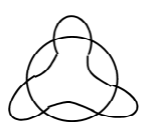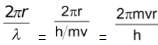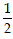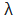# Derivation Of Angular Momentum From De Broglie Equation

## Atomic Structure of Class 11

According to Bohr’s model, the electron revolves around the nucleus in circular orbits. According to de Broglie concept, the electron is not only a particle but has a wave character also.

 If the wave is completely in phase, the circumference of the orbit must be equal to an integral multiple of wave length (λ) Therefore 2πr = nλ where ‘n’ is an integer and ‘r’ is the radius of the orbit But λ = h/mv ∴ 2πr = nh /mv (or) mvr = n h/2πwhich is Bohr’s postulate of angular momentum, where ‘n’ is the principal
quantum number.

“Thus, the number of waves an electron makes in a particular Bohr orbit in one complete revolution is equal to the principal quantum number of the orbit”.

Alternatively Number of waves ‘n’=where v and r are the velocity of electron and radius of that particular Bohr orbit in which number of waves are to be calculated, respectively.

The electron is revolving around the nucleus in a circular orbit. How many revolutions it can make in one second

Let the velocity of electron be v m/sec. The distance it has to travel for one revolution 2πr, (i.e., the circumference of the circle).

Thus, the number of revolutions per second is = V/2πr

Common unit of energy is electron volt which is amount of energy given when an electron is accelerated by a potential of exactly 1 volt. This energy equals the product of voltage and charge. Since in SI units coulombs × volts = Joules, 1 eV numerically equals the electronic charge except that joule replaces coulombs.

Illustration 7:Two particles A and B are in motion. If the wavelength associated with

[Distinction between the wave- particle nature of a photon and the particle- wave nature of a sub atomic particle]

 Photon Sub Atomic Particle 1. Energy = hν Energy =mv2 2. Wavelength =Wavelength = h/mv

[Note: We should never interchange any of the above]